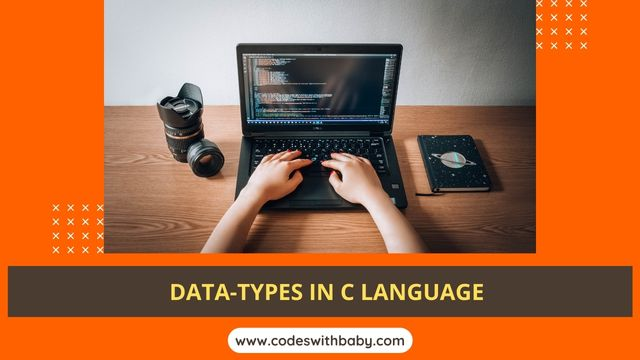# Data Types in C Programming Language With ExampleData Types in C Programming Language With Example

# Data Types in C Programming Language

Data Types: A Data type is a type of data. Data types can be used C Program. C language provides different data types. which can be used in the C language program. Data types provide in the c library.

Data types specify how we enter data into our programs and what type of data we enter. C language has some predefined set of data types to handle various kinds of data that we use in our program.

## Data types are two types in C Language

1. Pre-defined Data Types

2. User-defined Data Types or Derived Data Types.

Pre-defined Data Types: A pre-defined data type is also provided already define in the c Library. pre-defined data types like integer, floating and Character are pre-defined data types in C Language.

Example: 1.integer Type (int), 2.floating Type(float), 3.Character Type(char)

## User-defined Data Types or Derived Data Types

1. Pointer Types

2. Array

3. Structure

4. Union

## What data type does the C language have that C++ does not have?

These data types have different storage capacities. C language supports 2 different types of data types Primary data types and Derived data types.

Primary data types: These are fundamental data types in C namely integer (int), floating(float), character (char), and void.

Derived data types: Derived data types are arrays, functions, structures, and pointers. These are discussed in detail later.

 Primary Data Types Character Integer Float Void Char singed Unsigned float Signed Char int Int double Unsigned Char Short int Short int long

## Size and range of Integer type on a 16-bit machine.

 Type Size(bytes) Range Char or singed char 1 -128 to 127Range of signed character -128 to 127(-2^(n-1) to 2^(n-1) -1, where n is number of bits occupies by signed character 1bytes = 8bits ,- 2^(8-1) to 2^(8-1) -1- 2^(7) to 2^(7) -1- 128 to 127 unsigned char 1 0 to 255Range of unsigned character 0 to 255(0 to 2^(n) -1, where n is the number of bits occupies by unsigned character 1bytes = 8bits,0 to 2^(8) -10  to 2^(8) -10 to 256 - 10 to 255

## Integer Data Types C Language

Integer: Integers are used to store whole numbers. When a variable is declared without a short or long keyword the default is short–signed int.

### Size and range of Integer type on a 16-bit machine

 Type Size(bytes) Range int or signed int 2 -32,768 to 32767 (-2^(n-1) to 2^(n-1) -1, where n is number of bits occupies by signed character 1bytes = 8bits , - 2^(16-1) to 2^(16-1) -1 - 2^(15) to 2^(15) -1 -32,768   to 32767 unsigned int 2 0 to 65535 The range of unsigned integers is 0 to 65535 0 to 2^n - 1, where n is the number of bits occupied by unsigned integer 2bytes = 16 bits, 0 to 2^16 -1 so  2^16 -1 short int or signed short int 1 -128 to 127 -2^(n-1) to 2^(n-1) -1 Where n =  8 bit long int or signed long int 4 0 to 4,294,967,295 -2^(n-1) to 2^(n-1) -1, where n is the number of bits occupied by a long signed integer and is  4bytes = 32bits unsigned long int 4 0 to 4,294,967,295 -2^(n-1) to 2^(n-1) -1, where n is the number of bits occupied by a long signed integer and is  4bytes = 32bits

## Float Data Types C Language

Float: Float Data types are used to store real numbers.

### Size and Range of Float Type on 16-Bit Machine.

 Type Size(bytes) Range Float 4 3.4E-38 to 3.4E+38 Double 8 1.7E-308 to 1.7E+308 Long Double 10 3.4E-4932 to 1.1E+4932

## Void Data Types C Language

Void Data-Type: Void type means no value. This is usually used to specify the type of functions. For Example void main().

### Data Types And Their Control Strings

 Data_Type Size(bytes) Format String Char 1 %c Unsigned Char 1 %c Short or int 2 %i or %d Unsigned int 2 %u Long 4 %ld Unsigned Long 4 %lu Float 4 %f or %g Double 8 %lf Long Double 10 %lf Enum 2 %d

## Type Modifiers in C Programming Language

Type Modifiers: The keywords signed, unsigned, short and long are type modifiers. A type modifier changes the meaning of a basic data type and produces a new data type.

Each of these type modifiers applies to the basic data type int. The modifiers signed and unsigned are also applicable to the type char. The long-type modifier can be applicable to double types.

Example: Long, int, Unsigned Long int.

Write a Program to Declare Different Variables With Type Modifiers And Display

 #include#includevoid main(){short t =1;long k = 52111;unsigned u = 20;signed j = -1;clrscr();printf(“\n t =%d “,t);printf(“\n k = %ld”,k);printf(“\n u = %u”,u);printf(“\n j =%d”,j);} *****OUTPUT*****k = 54111n = 10j = -10